# Generalized functions, space of

distribution space

The space dual to the space of test (sufficiently good) functions. The Fréchet–Schwartz spaces (cf. Fréchet space) (type FS) and the spaces strongly dual to them (type DFS) play an important role here. A space of type FS is the projective limit of a directed set of Banach spaces and its dual is a space of type DFS. A space of type DFS is the inductive limit of a directed set of Banach spaces and its dual is a space of type FS. Spaces of types FS and DFS are complete, separable, reflexive, and Montel. In spaces of types FS and DFS weak and strong convergence coincide.

## Contents

### Examples of spaces of test and generalized functions.

1) The spacesand. The space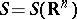of (rapidly-decreasing) test functions consists of the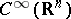-functions that together with all their derivatives decrease at infinity faster than any power of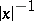. This space is the projective limit of the sequence of Banach spaces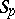,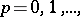consisting of the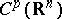-functions with norm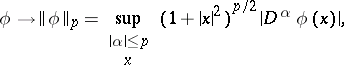and the inclusionis compact;is of type FS. The dual space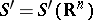(the space of generalized functions of slow growth) is the inductive limit of the sequence of Banach spaces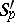, where the imbedding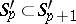is compact, so that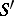is of type DFS. If a sequence of generalized functions is (weakly) convergent in, then it converges with respect to the norm of functionals in some. The Fourier transformation is an isomorphism on the spacesand.

2) The spaces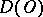and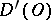(an open set in). The space of test functions consists of the-functions that have compact support in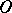(see Support of a generalized function). It is endowed with the topology of the strong inductive limit of the (increasing) sequence of spaces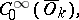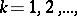of type FS, where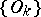is a strictly-increasing sequence of open sets that exhausts,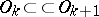,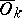compact,. The space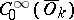is the projective limit of the (decreasing) sequence of Banach spaces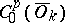,consisting of thefunctions with support inand with normwhere the imbeddingis compact. Letbe the space (strongly) dual to;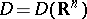and. A sequence of test functions inconverges inif it converges in some space. A sequence of generalized functions inconverges inif it converges on every element of(weak convergence). For a linear functionalonto be a generalized function init is necessary and sufficient that for any open set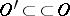there exist numbersandsuch that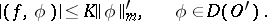The spaceis (weakly) complete: If a sequence of generalized functions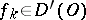,is such that for anyinthe sequence of numbersconverges, then the functional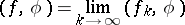belongs to. A generalized function inhas unrestricted "growth" in a neighbourhood of the boundary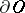; in particular, any function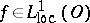determines a generalized function inby the formula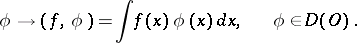3) The spacesand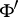. Let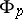be the Banach space of all functions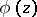,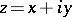, that are holomorphic in the tubular neighbourhood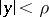,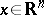, with norm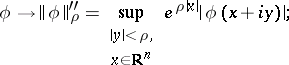the imbedding,, is compact. Letbe the inductive limit of the (increasing) sequence of spaces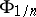,. The spaceis of type DFS, and its dualis of type FS. The elements ofare Fourier hyperfunctions;is also isomorphic to the space.